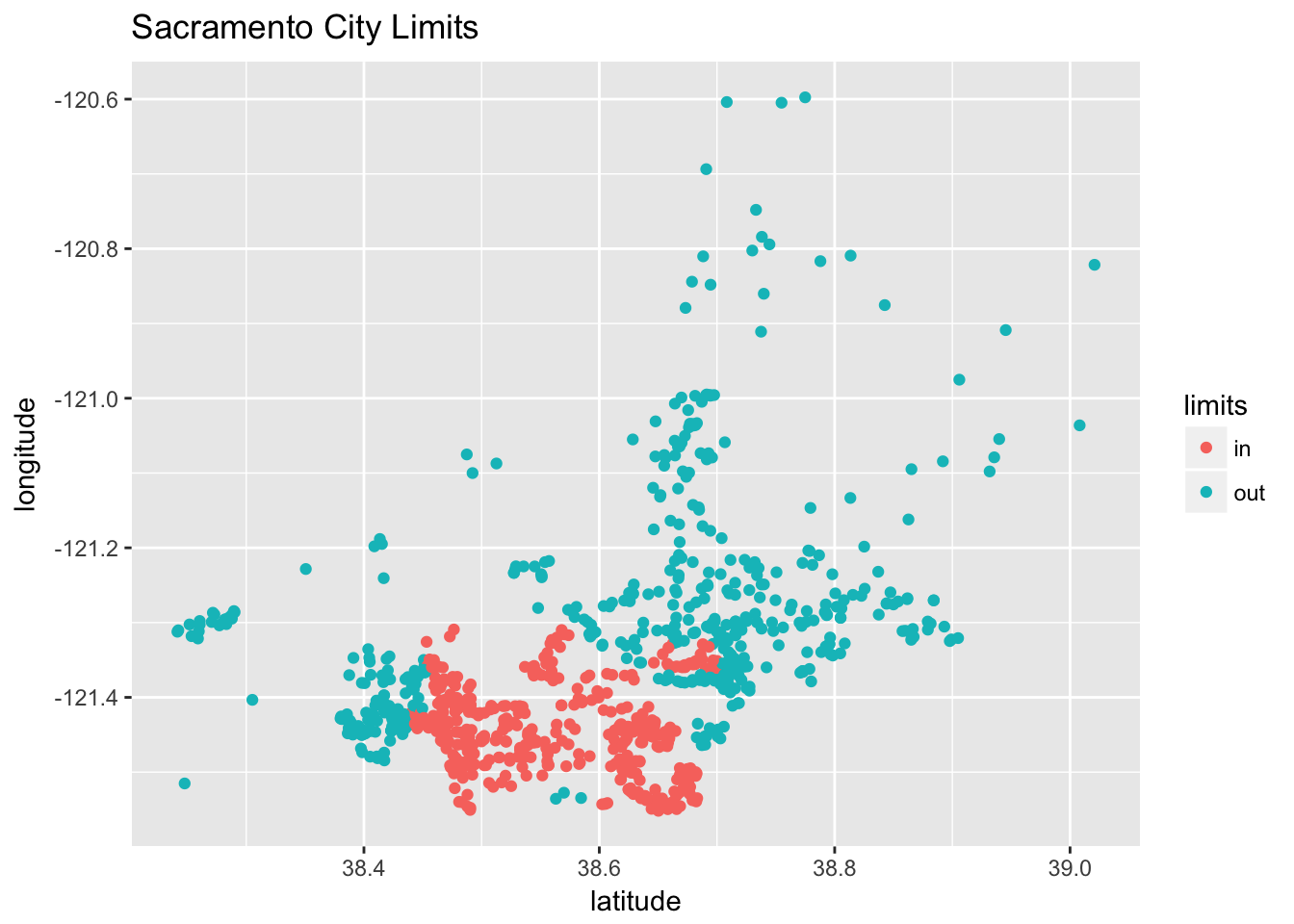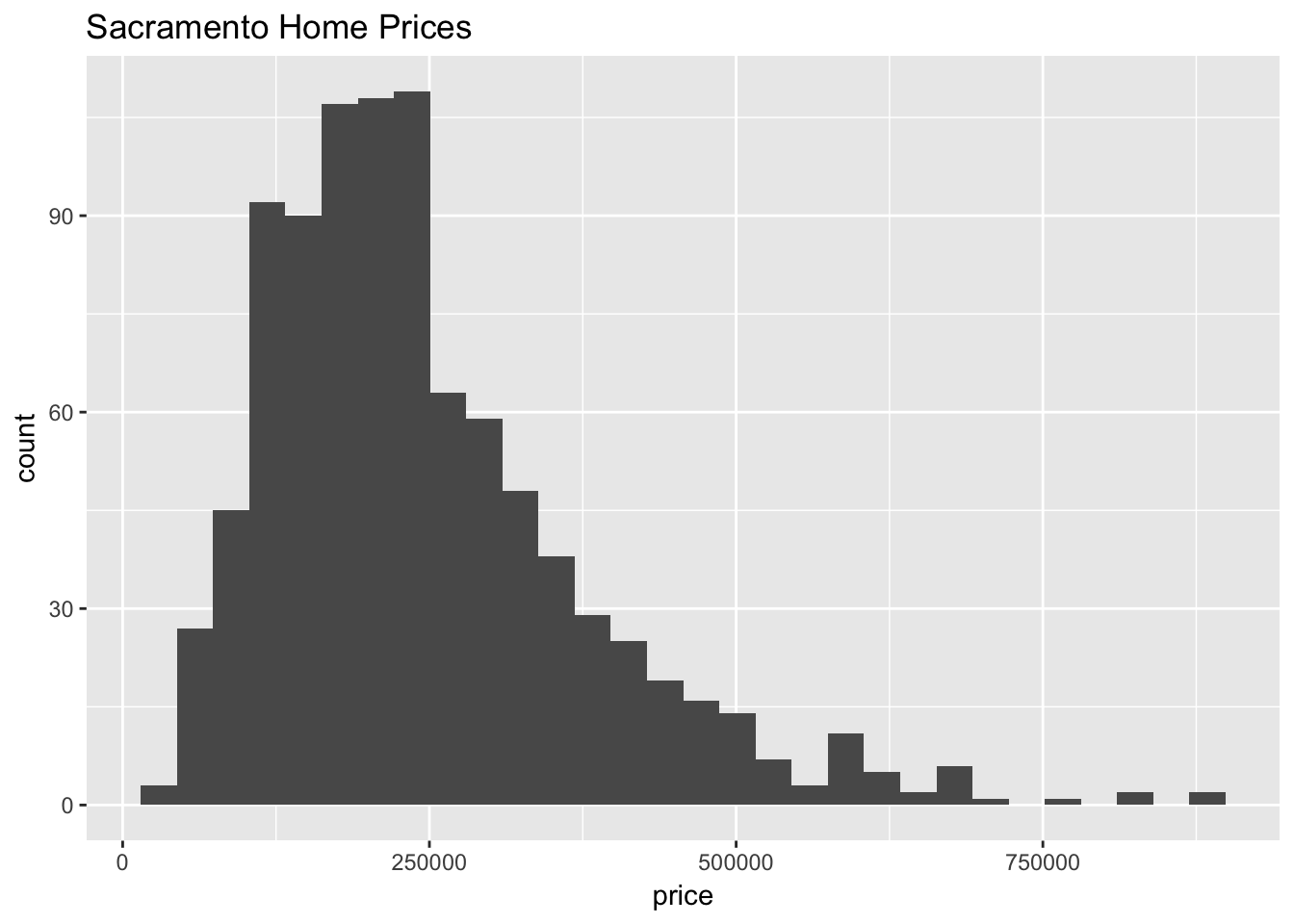Please see the homework policy document for detailed instructions and some grading notes. Failure to follow instructions will result in point reductions.

“How did it get so late so soon?”

Dr. Seuss

For this homework we will again use the Sacramento data from the caret package. You should read the documentation for this data. The goal of our modeling will be to predict home prices.

You may only use the following packages:

library(caret)
library(randomForest)
library(tidyverse)
library(knitr)
library(kableExtra)

Before modeling, we will perform some data preparation.

Instead of using the city or zip variables that exist in the dataset, we will simply create a variable indicating whether or not a house is technically within the city limits Sacramento. (We do this because they would both be factor variables with a large number of factors. This is a choice that is made due to laziness, not because it is justified. Think about what issues these variables might cause.)

data(Sacramento)
sac_data = Sacramento
sac_data$limits = factor(ifelse(sac_data$city == "SACRAMENTO", "in", "out"))
sac_data = subset(sac_data, select = -c(city, zip))

A plot of longitude versus latitude gives us a sense of where the city limits are.

qplot(y = longitude, x = latitude, data = sac_data,
col = limits, main = "Sacramento City Limits ")You should consider performing some additional exploratory data analysis, but we provide a histogram of the home prices.

qplot(x = price, data = sac_data, main = "Sacramento Home Prices")After these modifications, we test-train split the data.

set.seed(42)
sac_trn_idx  = sample(nrow(sac_data), size = trunc(0.80 * nrow(sac_data)))
sac_trn_data = sac_data[sac_trn_idx, ]
sac_tst_data = sac_data[-sac_trn_idx, ]

The training data should be used for all model fitting. Do not modify the data for any exercise in this assignment.

## Exercise 1 ($$k$$-Nearest Neighbors Preprocessing)

For this exercise, we will create $$k$$-nearest neighbors models in an attempt to be able to predict price. Do not modify sac_trn_data. Do not use the baths variable. Use the knnreg function from the caret package.

Consider three different preprocessing setups:

• Setup 1
• Numeric variables not scaled.
• Factor variables remain factors. knnreg() will use one-hot encoding.
• Setup 2
• Numeric variables are scaled to have mean 0 and standard deviation 1.
• Factor variables remain factors. knnreg() will use one-hot encoding.
• Setup 3
• Numeric variables are scaled to have mean 0 and standard deviation 1.
• Factor variables coerced to numeric. (We could then scale them, but for simplicity we will not.)

For each setup, train models using values of k from 1 to 100. For each, calculate test RMSE. (In total you will calculate 300 test RMSEs, 100 for each setup.) Summarize these results in a single plot which plots the test RMSE as a function of k. (The plot will have three “curves,” one for each setup.) Your plot should be reasonably visually appealing, well-labeled, and include a legend.

## Exercise 2 (Comparing Models)

For this exercise, we will create two additional models which we will compare to the best $$k$$-nearest neighbors model from the previous exercise. Again, do not modify sac_trn_data and do not use the baths variable.

Fit:

• A linear model using the formula: price ~ . + sqft:type + type:limits - baths
• A random forest that using all available predictors, excluding baths.

Create a well-formatted markdown table that displays the test RMSEs for these two models, as well as the best model from the previous exercise.

## Exercise 3 (Visualizing Results)

For each of the models in Exercise 2, create a Predicted vs Actual plot. Each plot should:

• Plot the predicted value ($$y$$-axis) versus the actual value in the test set ($$x$$-axis).
• Include a line indicating where $$x = y$$.
• Use a title indicating the model results being visualizing.
• Include some modification of default behavior to make the plot more visually appealing.

Arrange the three plots side-by-side in a single row.

## Exercise 4 (Test-Train Split)

Repeat Exercise 1, but with the following train and test data. Again, summarize your results in a plot.

set.seed(432)
sac_trn_idx_new  = sample(nrow(sac_data), size = trunc(0.80 * nrow(sac_data)))
sac_trn_data_new = sac_data[sac_trn_idx_new, ]
sac_tst_data_new = sac_data[-sac_trn_idx_new, ]

## Exercise 5 (Concept Checks)

[a] Which of the 300 models trained in Exercise 1 do you feel performs best?

[b] Based on your results for Exercise 1, do you feel that scaling the numeric variables was appropriate?

[c] Based on your results for Exercise 1, do you feel that the method used to utilize the factor variables had a large effect?

[d] Based on your results for Exercise 2, which of these models do you prefer?

[e] Based on your results for Exercise 3, do you prefer a different model than part [d]? If so which and why?

[f] Based on your results for Exercise 4, do you prefer a different preprossesing setup for $$k$$-nearest neighbors? (Compared to your preference based on Exercise 1.) If so, which and why?

[g] Based on your results for Exercise 4, do you prefer a different value of $$k$$ for $$k$$-nearest neighbors? (Compared to your preference based on Exercise 1.) If so, which and why?